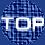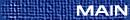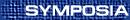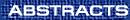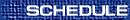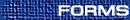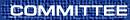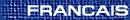Scattering Theory and Integrable Systems / Diffusion inverse et systèmes intégrables
(Jacek Szmigielski, Organizer)

MARK ADLER, Department of Mathematics, Brandeis University, Waltham, Massachusetts  02454, USA
Generalized orthogonal polynomials, integral mechanics and Darboux transforms on band matrices and weights

Classically, a single weight on an interval of the real line leads to moments, orthogonal polynomials and tridiagonal matrices. Appropriatedly deforming this weight with times t=(t1,t2,...), leads to standard Toda lattice and t-functions, expressed as hermitian matrix integrals. This talk is concerned with a sequence of t-perturbed weights, rather than one single weight.One example is m-periodic sequences of weights leading to 2m+1 band matrices of recurrence relations among generalized orthogonal polynomials and we use the associated integrable system, 2-Toda, to perform a Darboux transform on the matrix and the weights.

RICHARD BEALS, Yale University, New Haven, Connecticut  06520-8283, USA
Inverse scattering, strings, and moments

Work with David Sattinger and Jacek Szmigielski is described. A number of finite-dimensional integrable systems can be integrated explicitly by converting them to problems for (possibly indefinite) string densities. These include the multi-peakon/antipeakon cases of the Camassa-Holm equation, the finite-dimensional reduction of the Hunter-Saxton equation, and flows of Jacobi matrices, such as the finite Toda lattice. In each case the Weyl function for the string problem has a partial fraction expansion that incorporates the scattering data and a continued fraction expansion that incorporates the string data. A classical result of Sttieltjes recovers the latter data from the former. In particular, asymptotic phase shifts are easily computed.

YURI BEREST - I, Department of Mathematics, Cornell University, Ithaca, New York  14853-4201, USA

The question of characterizing linear differential systems which propagate waves without diffusion (known also as the problem of lacunas) goes back to the classical work of Hadamard and Petrovsky. In early 70s Atiyah, Bott and Garding developed a profound and nearly complete theory of lacunas for hyperbolic operators with constant coefficients. Extending this theory to operators with variable coefficients presents a major challenge, and the problem of lacunas still remains largely open in this general case. The talk will review some recent results and ideas in this direction.

YURI BEREST - II, Department of Mathematics, Cornell University, Ithaca, New York  14853-4201, USA
Geometry without points

In this talk I will explain connections between four apparently unrelated objects:

(1) Hilbert schemes of points on the complex affine plane,
(2) Ideal class spaces of the first complex Weyl algebra,
(3) Calogero-Moser Hamiltonian systems,
(4) The Adelic Grassmannian introduced by G. Wilson.
>From the perspective of integrable systems, an (apparently new) observation is that the space of algebraic solutions to the KP hierarchy can be identified with the moduli spaces of framed line bundles (or, more precisely, rank one torsion-free coherent sheaves) on M. Artin's quantum projective plane (somewhat in parallel with the well-known ADHM construction of instanton solutions to the classical Yang-Mills equations).

PERCY DEIFT, Courant Institute and the University of Pennsylvania
On the integrable method and some recent examples of its unreasonable effectiveness

Recent applications of the ``Integrable Method'' to fields as far apart as random matrix theory, orthogonal polynomials, combinatorics and statistical growth models, are rooted in the ideas introduced by Gardner, Greene Kruskal and Miura in their famous solution of the KdV equation in 1967. The speaker plans to trace these developments and to discuss some of the above applications.

MISHA GEKHTMAN, University of Notre Dame, Notre Dame, Indiana  46556-5683, USA
Inverse moment problem for elementary co-adjoint orbits

We give a solution to the inverse moment problem for a class of Hessenberg and symmetric matrices related to integrable lattices of Toda type. (Joint work with L. Faybusovich)

MARIA GOLENISHCHEVA-KUTUZOVA, University of Florida, Department of Mathematics, Gainesville, Florida  32611-8105, USA
Soliton equations in terms of intertwining operator

The integrability of a large classes of nonlinear systems and even of the hierarchies of integrable systems (including the famous KP, KdV and NLS hierarchies) is related to a big group of hidden symmetries. First this approach to the KP hierarchy appeared in the paper of M. Sato and then was developed in the papers of Kyoto school, using the boson-fermion correspondence in 2-dim QFT. They interpret the set of solutions of the KP-hierarschy as an orbit of the vacuum vector of the group GLinfty on the infinite-dimentional Grassmanian. We propose a unified method of constructing the hierarchies of integrable system with a given group of hidden symmetries in terms of intertwining operators. This is a very beautiful application of representation theory of infinite dimensional Lie groups.

JACQUES HURTUBISE, Department of Mathematics and Statistics, McGill University, Montréal, Québec  H3A 2K6
Multi-Hamiltonian structures, classical r-matrix systems spectral transforms and separation of variables

Classical r-matrices of rational, trigonometric and elliptic type may be used to define polynomial families of multi-Hamiltonian structures on loop algebras and loop groups corresponding, respectively, to linear (Lie-Poisson) and quadratic (Poisson-Lie/Sklyanin) brackets. The standard r-matrix theory implies commutativity and complete integrability of the flows generated by spectral invariants on finite dimensional Poisson submanifolds consisting of meromorphic Lax matrices over the base curve (of genus 0 or 1) with given pole divisor. The spectral transform yields an identification with the space consisting of pairs of: spectral curves and sheaves supported on them, on which a natural family of algebro-geometric Poisson structures is defined. A third way to view such Poisson spaces is by identification with symmetric products of a holomorphic Poisson surface with itself, leading to a separation of variables of the flows generated by spectral invariants in the associated ``spectral Darboux coordinates''. It is shown that the generalized Gel'fand-Zakharevich commuting invariants associated to the multi-Hamiltonian structures are the same as the spectral invariants derived from the r-matrix theory, and the ``Nijenhuis-Darboux'' coordinates given by the eigenvalues and eigenvectors of the Nijenhuis tensor coincide with the spectral Darboux coordinates. Examples illustrating these results include: constrained oscillators in n-dimensions, classical spin systems, Toda and Volterra lattices and reduced systems associated with commuting flows on the stationary manifolds of the NLS, KdV and Boussinesq hierarchies.

GERARD MISIOLEK, University of Notre Dame
Well-posedness results for an integrable evolution equation

I will discuss recent results on the periodic initial value problem for the Camassa-Holm equation. I will review the connection with the Bott-Virasoro group and describe how it can be used to derive sharp results on local well-posedness of the corresponding Cauchy problem.

YOSHIMASA NAKAMURA, Graduate School of Informatics, Kyoto University, Kyoto City  606-8501, Japan
Solving integrable systems in terms of continued fractions

In his fundamental paper by J. Moser (1975) an inverse scattering problem for the finite nonperiodic Toda equation was shown to be solved by using the Chebyshev continued fraction (C-fraction). The discrete time Toda equation gives a method for computing the C-fraction from a given power series. Recently, the C-fraction is successfully applied to the Cammassa-Holm equation by Beals, Sattinger and Szmigielski. In this talk I will discuss the Schur flow and the relativistic Toda equation in terms of the T-fraction which includes the Perron continued fraction. Integrable discretizations of these integrable systems help me to design new algorithms for computing the T-fraction. A joint work with Y. Minesaki and A. Mukaihira.

BEATRICE PELLONI, Department of Mathematics, University of Reading, Reading  RG6 6AX, United Kingdom
Applications of the Fokas transform method to the study of boundary value problems for PDEs in two dimensions

We survey some recent results obtained by using the method for solving initial boundary value problems recently introduced by Fokas; see the review in .

The Fokas transform method is a general method for analyzing boundary value problems for integrable PDE's in two dimensions. An equation in two dimensions is called integrable if it admits a Lax pair formulation, i.e. if it can be written as the compatibility condition of two linear eigenvalue equations. Examples of such equations are linear PDEs with constant coefficients and the usual nonlinear integrable PDEs such as the nonlinear Schrödinger equation (NLS). The Fokas method, in the two dimensional case, is based on performing the simultaneous spectral analysis of these two equations.

Assuming the existence of a solution of a given boundary value problem, this yields: (1)  an integral representation of the solution in Ehrenpreis form, namely a representation with explicit dependence on the two equation variables; (2)  a global relation between the boundary values of the solution.

Moreover, by exploiting the invariance properties of the global relation of (2), it is possible to identify the boundary value problems that are well posed, and prove rigorous existence results for the solution of such problems.

This method has been so far extensively applied to polygonal domains. The cases considered include linear evolution equations with spatial derivatives of arbitrary order on the half line , on a finite interval , and in time-dependent domains , the NLS on the half line  and on a finite interval , other nonlinear integrable PDEs [7, 13], and several elliptic PDEs [6, 1]. Recently, progress has been made on extending the method to more dimensions.

The author and Fokas have applied this method in the case of integrable evolution equations in one space dimension, on a finite interval or in a time-dependent domain. The aim of this presentation is to discuss the Fokas transform method in general and to give the precise results obtained when this method is applied to the above two cases.

 References

1. D. ben-Avraham and A.S. Fokas, The solution of the modified Helmholtz equation in a wedge and an application to diffusion-limited coalescence. Phys. Lett. A 263(1999).
2. A.S. Fokas, On the integrability of linear and nonlinear PDEs. J. Math. Phys. 41(2000), 4188.
3. A.S. Fokas and A.R. Its, The generalised Ehrenpreis-Palamodov representation and the long time asymptotics of evolution equations. 2000, preprint.
4.      , The Nonlinear Schrödinger equation on a finite interval, 2000, preprint.
5. A.S. Fokas, A.R. Its and L.Y. Sung, The Nonlinear Schrödinger equation on the half line. 2000, preprint.
6. A.S. Fokas and A. Kapaev, An integral transform method for the Laplace equation in a polygon. 2000, preprint.
7. A.S. Fokas and C.R. Menyuk, Integrability and similarity in transient stimulated Raman scattering. J. Nonlin. Science 9(1999), 1-31.
8. A.S. Fokas and B. Pelloni, A method of solving moving boundary value problems for linear evolution equations. Phys. Rev. Lett. 84(2000), 4785.
9.      , Two-point boundary value problems for linear evolution equations. Math. Proc. Cambridge Philos. Soc. (2000), in press.
10.      , Integrable evolution equations in time-dependent domains. Inv. Prob. (2000), in press.
11.      , Boundary value problems for linearized Boussinesq type systems. Proceedings of the 5th International conference on mathematical and numerical aspects of wave propagation, Santiago de Compostela, Spain, July 10-14, 2000.
12. A.S. Fokas and L.Y. Sung, Initial boundary value problems for linear dispersive evolution equations on the half-line. 1999, preprint.
13. A.S. Fokas, D. Tsoubelis and L.Y. Sung, The inverse spectral method for colliding gravitational waves. Math. Phys. Anal. Geom. 1(1999), 313-330.
14. P.C. Sabatier, Elbow scattering and inverse scattering applications to LKdV and KdV. J. Math. Phys. 41(2000), 414.

ENRIQUE REYES, Department of Mathematics, Yale University, New Haven, Connecticut  06520, USA
On differential geometry and integrability

We survey some recent developments in the geometrical study of nonlinear partial differential equations:

We introduce the class of equations of pseudo-spherical type, and show that their basic properties can be understood in geometrical terms. We will consider mainly their conservation laws, and the existence of Backlund/linearizing transformations. We will also define hierarchies of equations describing pseudo-spherical surfaces, and present transformations between any two hierarchies of pseudo-spherical type.

Finally, we apply this theory to the study of the Camassa-Holm shallow water equation. In particular, we will show how to construct its conservation laws.

DAVID SATTINGER, Department of Mathematics and Statistics, Utah State University, Logan, Utah  84322, USA
Solitary wave interactions for the Euler-Poisson equations

Experimental studies on interacting solitary waves of the Euler-Poisson equations for ion acoustic waves in plasmas were carried out at a range of velocities. It is found that even at high Mach numbers, the interactions are highly elastic, producing trailing dispersive waves of very small order. At low amplitudes, the major discrepancy in the Korteweg-deVries approximation comes in the displacements of the emerging solitary waves from those of the 3-soliton solution, which are produced by resonant interactions between the solitary waves. Resonant interactions manifest themselves only at higher orders, so that the time of validity of the KdV approximation by itself is necessarily limited.

(Joint work with David Nicholls, University of Minnesota, and Mariana Haragus, University of Bordeaux.)

BARRY SIMON, Division of Physics, Mathematics and Astronomy, California Institute of Technology, Pasadena, California  91125-3700, USA
The A-function approach to inverse spectral theory

After reviewing the two approaches to the inverse problem for Jacobi matrices, I'll discuss their analogs for continuum Schrodinger operators focusing on an approach I introduced in Annals of Math. 150(1999), 1029-1057. This is the analog of continued fractions for the moment problem. Some open problems and conjectures will be discussed.